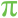# Pi

 Block Group: Constants Icon:The Pi block is a Number block. When the Pi block is created, the Pi block holds the mathematical constant pi.

For information on using dataflow blocks, see Dataflow.

## Input/Output Property

The following property of the Pi block can take input and give output.

• value (number)

value sets and returns the number held by this Pi block. When a Pi block is created, the value property holds the mathematical constant pi.

## Example

The following image demonstrates an example of the Pi block.